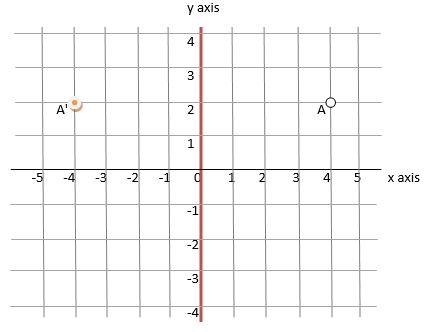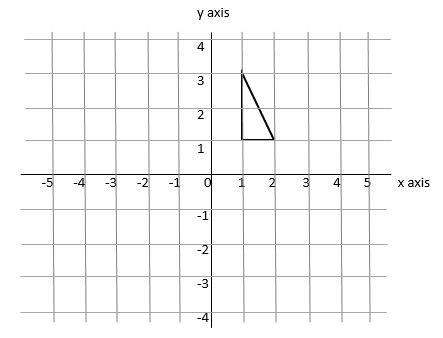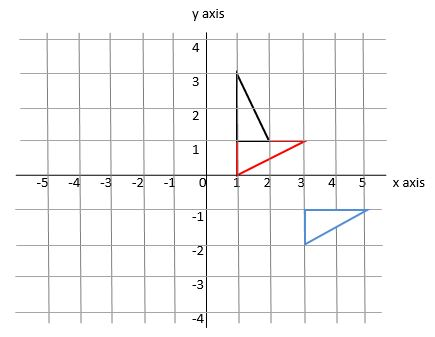# Reflections and Rotation

Reflections

Reflections are sometimes expected to be completed on triangular or similar paper which children will find unfamiliar, or they are to be carried out on a grid with co-ordinates.Let's look at the grid. The y axis will serve as our 'reflection line, but it could be any line that an examiner chooses. Make sure your child is careful to mark it on accurately if it isn't already marked on the diagram.

To reflect point A in the y-axis we must measure how far away from it it is, then put it an identical distance on the opposite side. The easiest way of doing this is to use a mirror placed on the reflection line, which is often provided, or to count the number of squares between the point and the line and measure them out from the reflection line to the new point.

In our example, 'A' is 4 squares to the right of the reflection line. Therefore, we must move it to the opposite (i.e. the left) of the reflection line. The new co-ordinates, which we'll call A', can be read off the grid as (-4, 2).

Although it is possible to work out the position of the new point mathematically, it's always simpler at this stage to simply count squares and put the new point on the grid.

Rotation

Rotation of a shape is not quite as straightforward as a translation; it requires spacial awareness to carry out effectively but there are mathematical things that you can do to work it out without physically moving an object around a grid. It is something that your child will be expected to do with squared paper (so they can see what's happening) or without, using common sense.

Let's look at an example and see what is expected.Rotate the black triangle 90° clockwise about the point (1, 1). We must think about the direction of movement - it is going clockwise. We should know what 90° looks like and be able to visualise any one of the individual lines moving through 90° or 180°. It is unlikely that other amounts of rotation will be asked for. Finally, we have to understand what 'around a point' means.

If you were to put a pin through a specific point (in this case, the co-ordinate (1, 1)) and rotate the shape around that, staying exactly the same distance away from that point if it isn't within the shape itself, you would be rotating the shape accurately.

So, we fix a virtual pin in the point (1, 1) and imagine it moving round a quarter turn (90°) clockwise. This takes the triangle round to the red triangle's position.The co-ordinates of the top point of the black triangle are (1, 3). The new point of the red triangle is at (3, 1). Note that one corner of the triangle is at (1, 1) in both. It is because this is the point of origin of the rotation, otherwise it would have changed.

Finally, look at the blue triangle. I have rotated this one 90° around (1, -1) to demonstrate that the point of rotation is very important - it gives a totally different final position. Note that each point of the black triangle is the same distance from the rotation point as the matching point of the blue triangle.

We use cookies to make your experience of our website better.

To comply with the new e-Privacy directive, we need to ask for your consent -